Exponents 2
Set 2 (10 Questions)
Determine the prime factors of all numbers through 50 and write the numbers as the product of their prime factors by using exponents to show multiples of a factor (e.g., 24 = 2 x 2 x 2 x 3 = two cubed times three)
From Mr. Anker Tests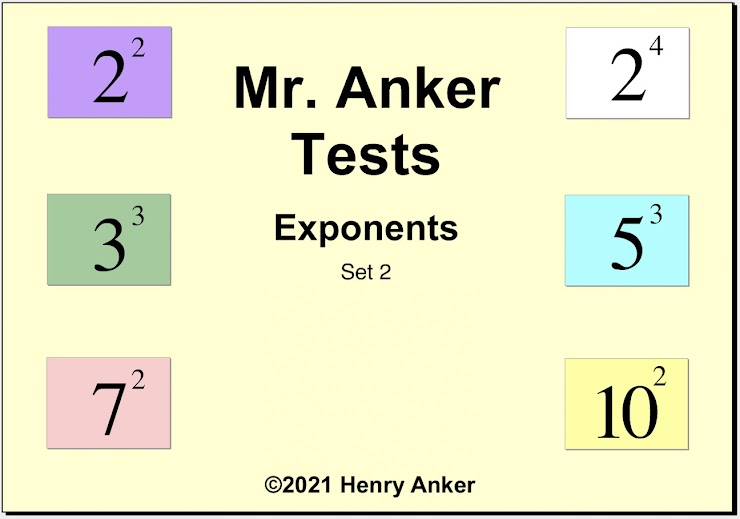Exponents Help Slide 1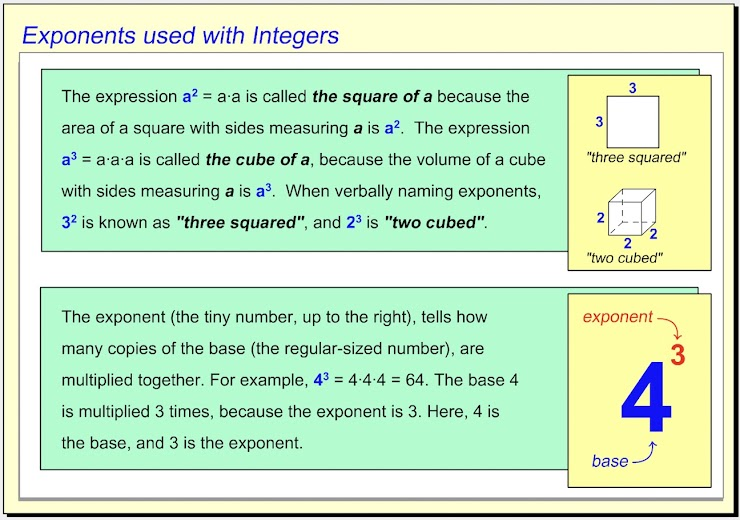Exponents Help Slide 2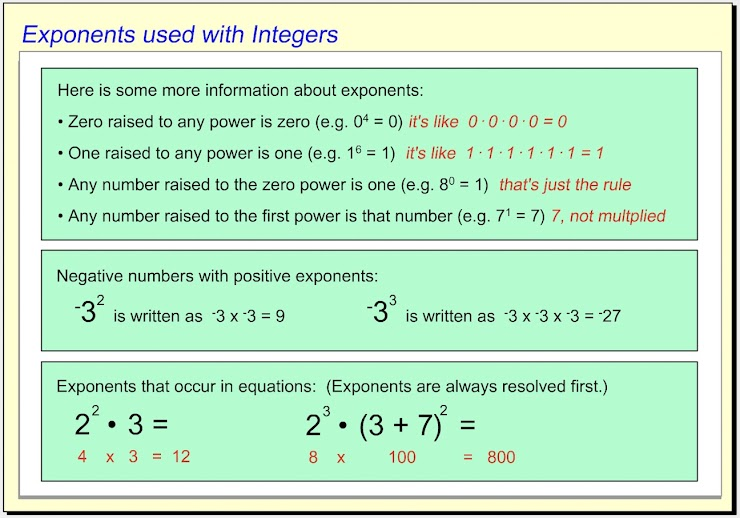Exponents Help Slide 3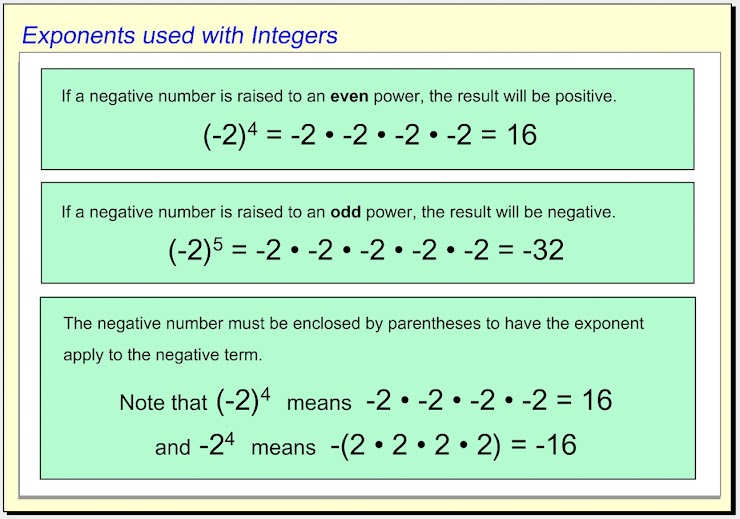1.  Which of the choices below is equal to the integer with the exponent shown? *
1 point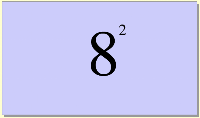2.  Use the dropdown menu to make this statement true:  "Eight squared is __________ four cubed." *
1 point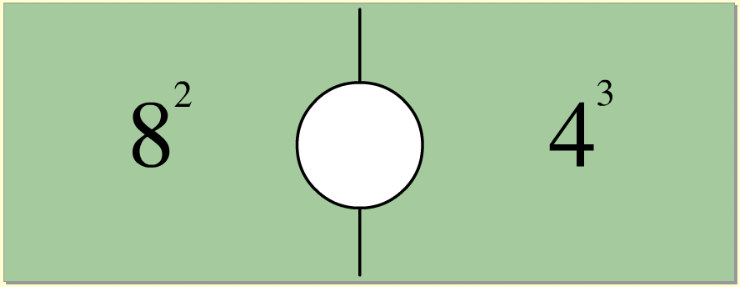3.  Which of the choices below is equal to the integer with the exponent shown? *
1 point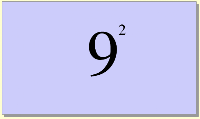4.  Use the dropdown menu to make this statement true:  "Five squared is __________ three cubed." *
1 point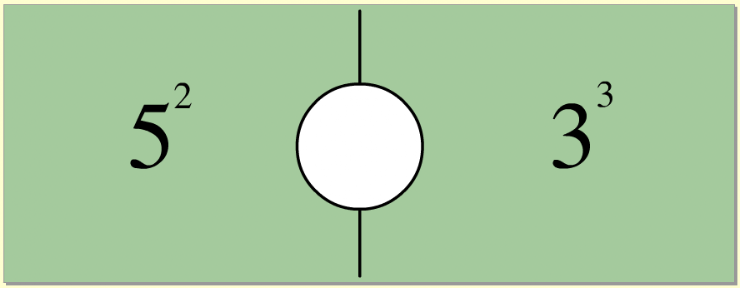5.  Which of the choices below is equal to the equation shown? *
1 point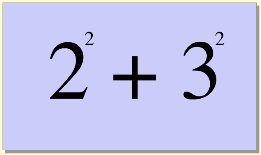6. Use the dropdown menu to make this statement true:  "Three cubed is ___________ two, raised to the fifth power." *
1 point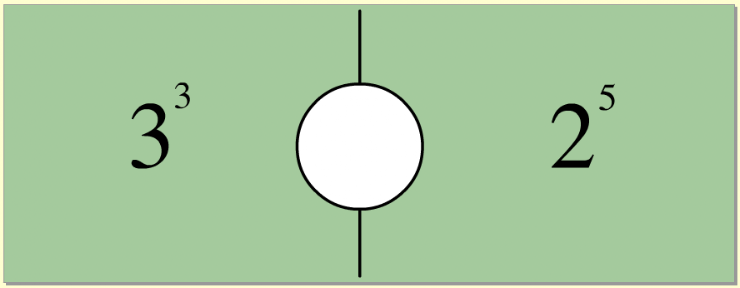7.  On the line below, enter the equal value as a whole number. *
1 point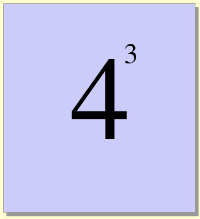8. Use the dropdown menu to make this statement true:  "Two, raised to the fourth power, is ___________ six squared." *
1 point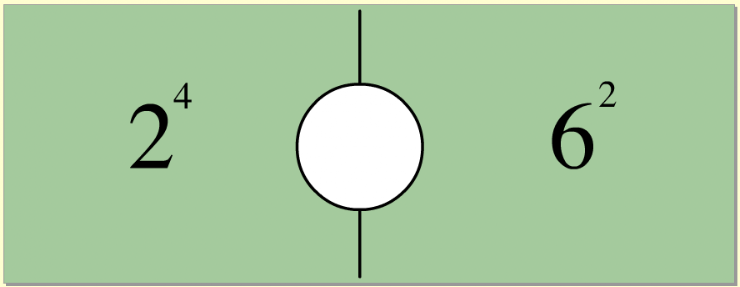9.  Which of the choices below is equal to the integer with the exponent shown? *
1 point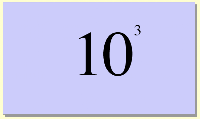10.   On the line below, enter the equal value as a whole number. *
1 point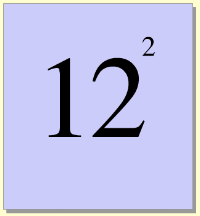Submit
Clear form